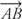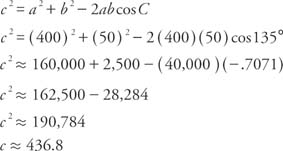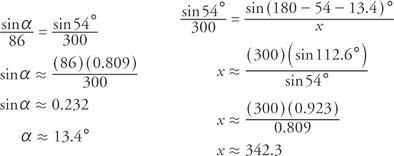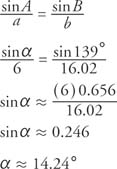## Vector Operations

In the physical world, some quantities, such as mass, length, age, and value, can be represented by only magnitude. Other quantities, such as speed and force, also involve direction. You can use vectors to represent those quantities that involve both magnitude and direction. One common use of vectors involves finding the actual speed and direction of an aircraft given its air speed and direction and the speed and direction of a tailwind. Another common use of vectors involves finding the resulting force on an object being acted upon by several separate forces.

Any quantity that has both size and direction is called a vector quantity. If A and B are two points that are located in a plane, the directed line segment from point A to point B is denoted by. Point A is the initial point, and point B is the terminal point.

A geometric vector is a quantity that can be represented by a directional line segment. From this point on, a vector will be denoted by a boldface letter, such as v or u. The magnitude of a vector is the length of the directed line segment. The magnitude is sometimes called the norm. Two vectors have the same direction if they are parallel and point in the same direction. Two vectors have opposite directions if they are parallel and point in opposite directions. A vector that has no magnitude and points in any direction is called the zero vector. Two vectors are said to be equivalent vectors if they have the same magnitude and same direction.

Figure 1 demonstrates vector addition using the tail‐tip rule. To add vectors v and u, translate vector u so that the initial point of u is at the terminal point of v. The resulting vector from the initial point of v to the terminal point of u is the vector v + u and is called the resultant. The vectors v and u are called the components of the vector v + u. If the two vectors to be added are not parallel, then the parallelogram rule can also be used. In this case, the initial points of the vectors are the same, and the resultant is the diagonal of the parallelogram formed by using the two vectors as adjacent sides of the parallelogram.Figure 1

In order to multiply a vector u by a real number q, multiply the length of u by | q | and reverse the direction of u if q < 0. This is called scalar multiplication. If a vector u is multiplied by −1, the resulting vector is designated as − u. It has the same magnitude as u but opposite direction. Figure 2 demonstrates the use of scalars.Figure 2
Examples of vectors.

Example 1: A plane is traveling due west with an air speed of 400 miles per hour. There is a tailwind blowing in a southwest direction at 50 miles per hour. Draw a diagram that represents the plane's ground speed and direction (Figure  ).Figure 3
Drawing for Example 1— vector representation.

The vector represented in the preceding example is known as a velocity vector. The bearing of a vector v is the angle measured clockwise from due north to v. In the example, the bearing of the plane is 270° and the bearing of the wind is 225°. Redrawing the figure as a triangle using the tail‐tip rule, the length (ground speed of the plane) and bearing of the resultant can be calculated (Figure  4).Figure 4
Drawing for Example 1— angle representation.

First, use the law of cosines to find the magnitude of the resultant.Then, use the law of sines to find the bearing.The bearing, β, is therefore 270° − 4.64°, or approximately 265.4°.

Example 2: A plane flies at 300 miles per hour. There is a wind blowing out of the southeast at 86 miles per hour with a bearing of 320°. At what bearing must the plane head in order to have a true bearing (relative to the ground) of 14°? What will be the plane's groundspeed (Figure  5)?Figure 5
Drawing for Example 2.

Use the law of sines to calculate the bearing and the groundspeed. Because these alternate interior angles are congruent, the 54° angle is the sum of the 14° angle and the 40° angle.Therefore, the bearing of the plane should be 14° + 13.4° = 27.4°. The groundspeed of the plane is 342.3 miles per hour.

Any vector can be broken down into two component vectors, a horizontal component and a vertical component. These component vectors are called projections (Figure  6).Figure 6
Example of projections.Example 3: A force of 11 pounds and a force of 6 pounds act on an object at an angle of 41° with respect to one another. What is the magnitude of the resultant force, and what angle does the resultant force form with the 11‐pound force (Figure  7)?Figure 7
Drawing for Example 3.

First, use the Law of Cosines to find the magnitude of the resultant force.Next, use the Law of Sines.Thus the resultant force is 16.02 pounds, and this force makes an angle of 14.24° with the 11‐pound force.